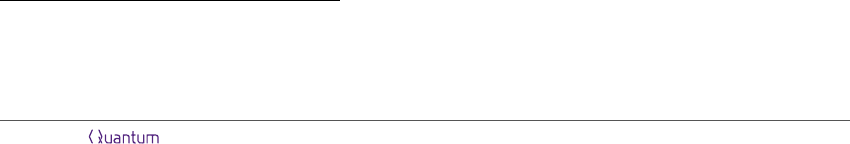Quantum query complexity of symmetric oracle
problems.
Daniel Copeland
1
and Jamie Pommersheim
2
1
UC San Diego
2
Reed College
We study the query complexity of quantum learning problems in which the oracles
form a group G of unitary matrices. In the simplest case, one wishes to identify the
oracle, and we ﬁnd a description of the optimal success probability of a t-query quantum
algorithm in terms of group characters. As an application, we show that Ω(n) queries
are required to identify a random permutation in S
n
. More generally, suppose H is
a ﬁxed subgroup of the group G of oracles, and given access to an oracle sampled
uniformly from G, we want to learn which coset of H the oracle belongs to. We call
this problem coset identiﬁcation and it generalizes a number of well-known quantum
algorithms including the Bernstein-Vazirani problem, the van Dam problem and ﬁnite
ﬁeld polynomial interpolation. We provide character-theoretic formulas for the optimal
success probability achieved by a t-query algorithm for this problem. One application
involves the Heisenberg group and provides a family of problems depending on n which
require n + 1 queries classically and only 1 query quantumly.
1 Introduction
An oracle problem is a learning task in which a learner tries to determine some information by
asking certain questions to a teacher, called an oracle. In our setting the learner is a quantum
computer and the oracle is an unknown unitary operator acting on some subsystem of the com-
puter. The computer asks questions by preparing states, subjecting them to the oracle, measuring
the results, and ﬁnally making a guess about the hidden information. How many queries to the
oracle are needed by the computer to guess the correct answer with high probability?
This paper addresses the following oracle problem. Fix a ﬁnite group G and a subgroup H G.
The elements of G are encoded as unitary operators by some unitary representation π : G U(V ).
Given oracle access to π(a) (for some unknown a G) the learner must guess which coset of H
the element a lies in. We focus on average case success probability, though an easy averaging ar-
gument, given in Section 2, shows that the worst case and average case query complexity are equal.
We call this problem coset identiﬁcation. This task encompasses many of previously studied
qauntum oracle problems, including univariate and multivariate polynomial interpolation over a
ﬁnite ﬁeld [CvDHS16, CCH18], the group summation problem [MP14, Zha15, BBC
+
01], and sym-
metric oracle discrimination [BCMP16]. In addition, the coset identiﬁcation problem we study gen-
eralizes the homomorphism evaluation problem for abelian groups studied by Zhandry in [Zha15],
which greatly inspired us. Section 7 gives details of this connection.
Daniel Copeland: daniel.copeland@gmail.com
Jamie Pommersheim: jamie@reed.edu
Accepted in Quantum 2021-03-03, click title to verify. Published under CC-BY 4.0. 1
arXiv:1812.09428v3 [cs.CC] 3 Mar 2021In this paper, we analyze the query complexity of the general coset identiﬁcation problem. We
prove that nonadaptive algorithms are optimal for any coset identiﬁcation problem. We provide
tools to reduce the analysis of query complexity to purely character theoretic questions (which
are themselves often combinatorial). In particular we derive a formula for the exact quantum
query complexity for coset identiﬁcation in terms of characters. In the case of symmetric oracle
discrimination (which itself includes polynomial interpolation as a special case) we ﬁnd the lower
and upper bound for bounded error query complexity.
Another motivation for our work is the study of nonabelian oracles. Much is known about
quantum speedups when the oracle is a standard Boolean oracle. Less is known about whether
oracle problems with nonabelian symmetries can oﬀer notable speedups. To that end we study the
follow scenario: suppose a group G acts by permutations on a ﬁnite set (we call a G-set). A
learner is given access to a machine which takes an element ω and returns a·ω for some hidden
group element a G. With as few queries as possible the learner should guess the hidden element
a G. The classical query complexity for this problem is a long-known invariant of G-sets called
the base size. For instance, if G is the full permutation group of Ω = {1, . . . , n} then n 1 queries
are required classically to determine the hidden permutation. This problem is a special case of
symmetric oracle discrimination and we can express the bounded error quantum query complexity
of this purely in terms of the character of the G-set . For instance, we ﬁnd that when G is the full
permutation group of X = {1, . . . , n} then n2
n+Θ(n
1/6
) queries are necessary (and suﬃcient)
to determine the hidden element.
This result bears some similarity to other work on learning problems related to the symmet-
ric group. Aaronson and Ambainis [AA14], who prove that at most a polynomial speedup can
be achieved in computing functions on n inputs which are invariant under the action of the full
symmetric group S
n
(using a standard evaluation oracle). Ben-David [BD16] proves that at most
a polynomial speedup is possible for Boolean functions deﬁned on the full symmetric group. More
recently, Dafni, Filmus, Lifshitz, Lindzay and Vinyals [DFL
+
21] have studied the query complex-
ity of Boolean functions deﬁned on the symmetric group, again proving a polynomial relationship
between the quantum and classical query complexities (as well as numerous other complexity mea-
sures). These results may be compared to the well-known fact that only polynomial speedups are
possible in computing total Boolean functions [BBC
+
01], the idea being that learning problems
on the full symmetric group correspond to total functions, while learning problems on a subgroup
correspond to partial functions. All of the results mentioned above are not directly comparable to
ours, since they use a standard evaluation oracle, while we examine a more symmetric “in-place”
oracle model.
The task of oracle identiﬁcation can be further reﬁned: ﬁx a group G, a G-set , and a func-
tion f : G X which is constant on left cosets of some subgroup H, and distinct on distinct
cosets. The (left) coset identiﬁcation problem is to determine f(a) given access to a permutational
black-box hiding a through the action on . For instance, when G = S
n
(the symmetric group),
Ω = {1, . . . , n} its deﬁning representation and f the sign homomorphism, it requires n 1 classical
queries to determine f(a). As a counterpoint to the harsh lower bound above we provide a family
of examples for this task parametrized by n in which the quantum query complexity is 1 while the
classical complexity is O(n). The groups we use are Heisenberg groups acting as small subgroups of
the full permutation group. This example is a nonabelian analogue of the fact that good quantum
speedups can be found in computing partial Boolean functions [BV97].
The paper is organized as follows. In section 2 we formalize coset identiﬁcation in the context
of quantum learning algorithms and review the notions of adaptive and nonadaptive learning. In
section 3 we prove that parallel queries suﬃce to produce an optimal algorithm for this task. Sec-
tion 4 applies this theorem to symmetric oracle discrimination and addresses numerous example
problems. In section 5 we return to the general coset identiﬁcation task and we prove the main
Accepted in Quantum 2021-03-03, click title to verify. Published under CC-BY 4.0. 2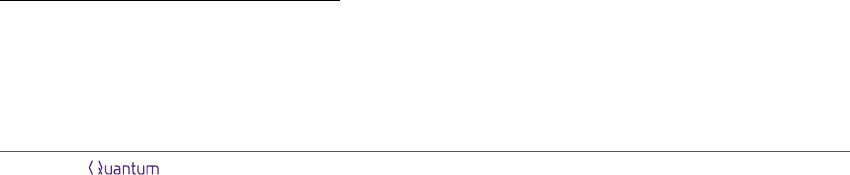theorem of this paper, Theorem 5.1, which is a formula for the success probability of an optimal t-
query algorithm in terms of characters. We use this in section 6 to compute the exact and bounded
error query complexity of some special examples (including the Heisenberg group example). We
conclude in section 7 by explaining how our work reproduces several previously known results
involving abelian oracles.
Our paper uses the language of representation theory of ﬁnite groups. A suitable reference is
the ﬁrst third of Serre’s textbook [Ser96]. We review some important notations later in Section
5 (in particular, the idea of induced representation is critical for the statement of our results.)
Here we mention that a representation of a ﬁnite group G always refers to a ﬁnite dimensional and
unitary representation of G over the complex numbers. In other words, a representation is a group
homomorphism π : G U (V ) (the unitary group of a f.d. vector space V ). We often think of V
as a left module for the group alegbra CG, and use the notation gv for π(g)v when the map π is
clear from the context.
2 Quantum learning from oracles
A quantum or classical oracle problem is described by a set of hidden information Y , a function
f : Y X (the function to learn or compute), and a representation of Y as operations on inputs
of some kind (which determines the oracles). Classically such a representation consists of a set of
inputs and an assignment taking each y Y to a permutation of , i.e. a map π : Y Sym(Ω).
A classical oracle problem is speciﬁed by a tuple (Y, , π, f). A classical computer has access to
π(y) for some unknown y Y by spending one query to input ω to learn π(y)·ω. The goal is to
determine f(y) with a high degree of certainty with as few queries as possible. More concretely, we
measure the eﬃcacy of an algorithm by its average case success probability, namely the probability
of correctly outputting f(y) supposing the hidden information y is sampled uniformly from Y . For
the highly symmetric problems considered in this paper, this is the same as the worst-case success
probability, as explained below.
The quantum representation of oracles is described by a Hilbert space V and an assignment
taking each y Y to a unitary operator of V , in other words a map π : Y U(V ). Thus a
quantum oracle problem is speciﬁed by a tuple (Y, V, π, f). The quantum computer spends one
query to input a state |ψi V to π(y) to acquire the state π(y)|ψi; the goal is to produce a state
and measurement scheme which outputs the value f(y).
Any classical oracle problem (Y, , π, f) determines a quantum oracle problem via linearization:
oracles will act on the Hilbert space C (spanned by the orthonormal basis {|ωi | ω }) by
permutation matrices.
We note that there are other oracle models used to encode permutations. One possibility is
to require an oracle to act on a bipartite system, with one subsystem speciﬁed to be the “input
register” and the other a “response register”.
1
While we do not speciﬁcally consider this model
here, we note that many oracle problems, such as polynomial interpolation and group summation,
that are normally formulated in this two-register setup do have an easy reformulation in our setup.
Thus, our results and analyses apply to these problems in their original two-register formulation.
See Section 7. However, in some cases, the two-register setup results in a set of oracles that do not
form a group, for instance in the work of Ambainis on permutation inversion [Amb02]. In general,
it is an interesting question (and to our knowledge, open) whether these oracle models are the
1
More precisely, one usually deﬁnes an abelian group structure on (usually cyclic) by deﬁning an operation
on Ω. Then the oracle hiding the permutation π(a) is deﬁned to act by |ω, bi 7→ |ω, (π(a) · ω) bi. Here ω, b Ω,
so both the input and response registers are copies of CΩ.
Accepted in Quantum 2021-03-03, click title to verify. Published under CC-BY 4.0. 3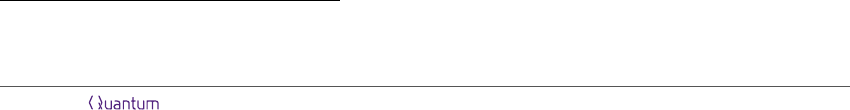same, or if they lead to diﬀerent query complexities.
2
A symmetric oracle problem is an oracle problem in which the hidden information is a group G
(so we are replacing Y with G) and the map π is a homomorphism G Sym(Ω) in the classical
case or G U(V ) in the quantum case. If π : G U (V ) is a homomorphism, then it is common
practice to regard V as a (left) CG-module where CG is the group algebra of G (spanned by an
orthonormal basis sometimes written without kets as {g | g G}. In module notation we some-
times write g · v := π(g)(v) (for g G, v V ) if the representation π is understood from context.
The quantum oracle arising from a symmetric classical problem is also symmetric.
Of special interest to us is the case when the function f to be learned is compatible with the
group structure G. An instance of the coset identiﬁcation problem is a symmetric oracle problem
(G, V, π, f) where the function f : G X is constant on left cosets of a subgroup H G and dis-
tinct on distinct cosets. We also assume f is onto. The typical example is when X = {gH | g G}
is the set of left cosets of H and f(g) = gH. An equivalent formulation is to say that X is a
transitive G-set and the map f : G X is a map of (left) G-sets (i.e., f(gh) = gf(h) for all
g, h G). Then the subgroup H can be recovered as the preimage of f(e).
For our analysis of the coset identiﬁcation problem, we focus on average case success probabil-
ity. The symmetry of the problem implies that worst case and average case success probabilites
are equal, as the following argument shows: provided an unknown oracle π(a) we can select g G
uniformly at random and preprocess our input by applying π(g). Then an optimal average-case
algorithm will return the coset containing ga with optimal average-case success probability. The
coset which contains a can then be retrieved by applying g
1
. Hence it suﬃces to consider the
average case success probability of any algorithm for this task (with the unknown oracle π(a) sam-
pled uniformly from G).
We examine bounded error and exact measures of query complexity. The exact (or zero error)
query complexity of a learning problem is the minimum number of queries needed by an algorithm
to compute f(y) with zero probability of error. The bounded error query complexity is the minimum
number of queries needed by an algorithm to compute f(y) with probability 2/3. The bounded
error query complexity is often studied for a family of problems growing with a parameter n and
so changing the constant 2/3 above to any number strictly greater than 1/2 will only change the
query complexity by a constant factor mostly ignored in asymptotic analysis.
Broadly speaking, there are two qualitatively diﬀerent approaches to solving an oracle prob-
lem. The ﬁrst approach is to ask questions one at a time, carefully changing your questions as you
copies of the teacher). This is known as using non-adaptive, or parallel queries.
Classically the adaptive model is at least as strong as the nonadaptive model, since you can
asking them one at a time). This is well-known to be true also in the quantum setting. In the next
section we will prove the converse for coset identiﬁcation:
Theorem 2.1. Suppose (G, V, π, f) describes an instance of coset identiﬁcation. Then there exists
a t-query quantum algorithm to determine f(a) with probability P if and only if there exists a
t-query nonadaptive query algorithm which does the same.
This theorem is certainly not true for arbitrary learning problems: Grover’s algorithm provides
2
As another modiﬁcation, one may propose that having access to an oracle O means an algorithm may choose
to access O or O
1
in any given query. This is a separate model which we do not consider here.
Accepted in Quantum 2021-03-03, click title to verify. Published under CC-BY 4.0. 4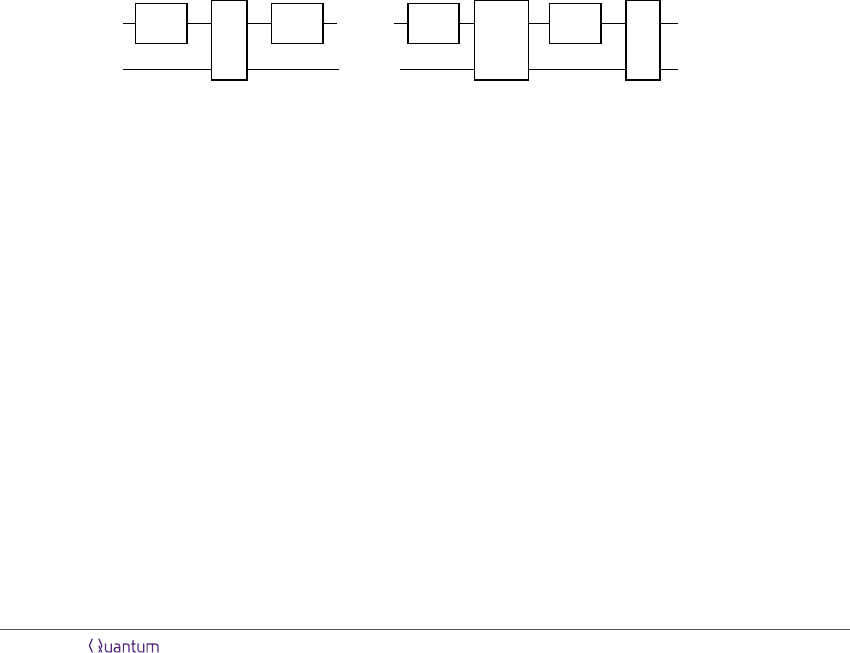an example in which any optimal algorithm must use adaptive queries [Zal99]. To prove the
Recall that a quantum learning problem is described by a tuple (Y, V, π, f : Y X) where Y
indexes the set of hidden information, V is a ﬁnite dimensional Hilbert space, π : Y U(V ) a
representation of the unknown information by unitary operators, and f is the function to learn.
The standard model for an adaptive algorithm is as follows (see e.g. [BBC
+
01, Section 3.2]):
A t-query adaptive quantum algorithm for the quantum oracle problem (Y, V, π, f : Y X)
consists of a tuple A = (N, ψ, {U
1
, . . . , U
t
}, {E
i
}) where
N is the dimension of the auxiliary workspace used in the computation
|ψi is a unit vector in V C
N
{U
1
, . . . , U
t
} is a set of unitary operators acting on V C
N
{E
x
}
xX
is a POVM with measurement outcomes indexed by X.
The algorithm uses t queries to the oracle π(a) (with a sampled uniformly from Y ) to produce
the output state
|ψ
A
a
i = U
t
(π(a) I)U
t1
(π(a) I) . . . (π(a) I)U
1
(π(a) I)|ψi
upon which the algorithm executes the measurement described by {E
x
}
xX
. Here and elsewhere
I denotes the identity operator (in this case acting on the space C
N
).
In quantum circuit notation the preparation of the state |ψ
A
a
|ψi
π(a)
U
1
π(a)
. . .
π(a)
U
t1
π(a)
U
t
= |ψ
A
a
i
By contrast, an algorithm is nonadaptive if at any point during the algorithm, the input for
some query does not depend on the results to any of the previous queries. Essentially this means
that all the inputs are completely determined before the algorithm begins. Classically, t nonadap-
tive queries are identical to t simultaneous queries to t copies of an oracle. This motivates the
following deﬁnition (cf [Mon10, Section 2]):
A t-query nonadaptive quantum algorithm for the oracle problem (Y, V, π, f) is a tuple A =
(N, ψ, {E
x
})
xX
where
N is the dimension of the auxiliary register.
|ψi is the input state, a unit vector of V
t
C
N
.
{E
x
} is a POVM indexed by X.
The algorithm operates on the input state to produce
|ψ
A
a
i = (π(a)
t
I)|ψi
which is then measured using the POVM {E
x
}. The next fact is very useful and follows immediately
from deﬁnitions.
Accepted in Quantum 2021-03-03, click title to verify. Published under CC-BY 4.0. 5Lemma 2.2. A t-query nonadaptive algorithm for the problem (Y, V, π, f) is the same as a single-
query nonadaptive algorithm for the oracle problem (Y, V
t
, π
t
, f).
The quantum circuit notation for the nonadaptive preparation of the state |ψ
A
a
i is drawn as
follows.
|ψi
π(a)
π(a)
.
.
.
π(a)
= |ψ
A
a
i
In either model, the algorithm A uses t copies of the unitary π(a) to produce a state |ψ
A
a
i.
Using the POVM {E
x
} results in a measurement value x X with probability
P (x | a) = hψ
A
a
|E
x
|ψ
A
a
i.
Since we assume the oracle is sampled uniformly from Y , the probability that A executes
successfully is
P
succ
(A) =
1
|Y |
X
aY
P (f(a) | a) =
1
|Y |
X
aY
hψ
A
a
|E
f(a)
|ψ
A
a
i.
2.2 Symmetric oracle problems
Suppose we have a symmetric oracle problem (G, V, π, f ). As mentioned in the introduction, since
we are focusing on query complexity and not on issues of implementation, analysis of this problem
depends only on the character χ
V
of π : G U(V ), as we prove in the lemma below. In fact, a
little more is true. Let Irr(G) denote the set of irreducible characters of G. Given a representation
π : G U(V ) deﬁne the set
I(V ) := {χ Irr(G) appearing in the representation V }
= {χ Irr(G) | (χ, χ
V
) > 0}.
Here we are using (·, ·) to denote the usual inner product of characters. If χ Irr(G) and (χ, χ
V
) >
0 we say that χ appears in the representation V .
Lemma 2.3. The optimal success probability of a t-query algorithm to solve a symmetric oracle
problem (G, V, π, f) depends only on I(V ) and f .
Proof. First, note that if U : V W is a Hilbert space isomorphism then we can deﬁne a new
oracle problem (G, W, UπU
1
, f) where the oracles now act on W . Any t-query algorithm to solve
the original problem can be “conjugated” by U (e.g. the input state |ψi becomes U|ψi and the
non-oracle unitaries and POVM are conjugated by U) to produce a t-query algorithm for the new
problem which succeeds with the same probability. Conversely any algorithm to solve the new
problem can be conjugated by U
1
to solve the old problem with the same probability. Therefore
oracle problems with isomorphic unitary representations of G will have the same t-query optimal
success probability. In other words, only the character χ
V
is relevant.
Second, we claim that the multiplicities of irreducible characters in V are not important; only
whether they appear in V or not. Indeed, adding a d-dimensional workspace to a computer’s
Accepted in Quantum 2021-03-03, click title to verify. Published under CC-BY 4.0. 6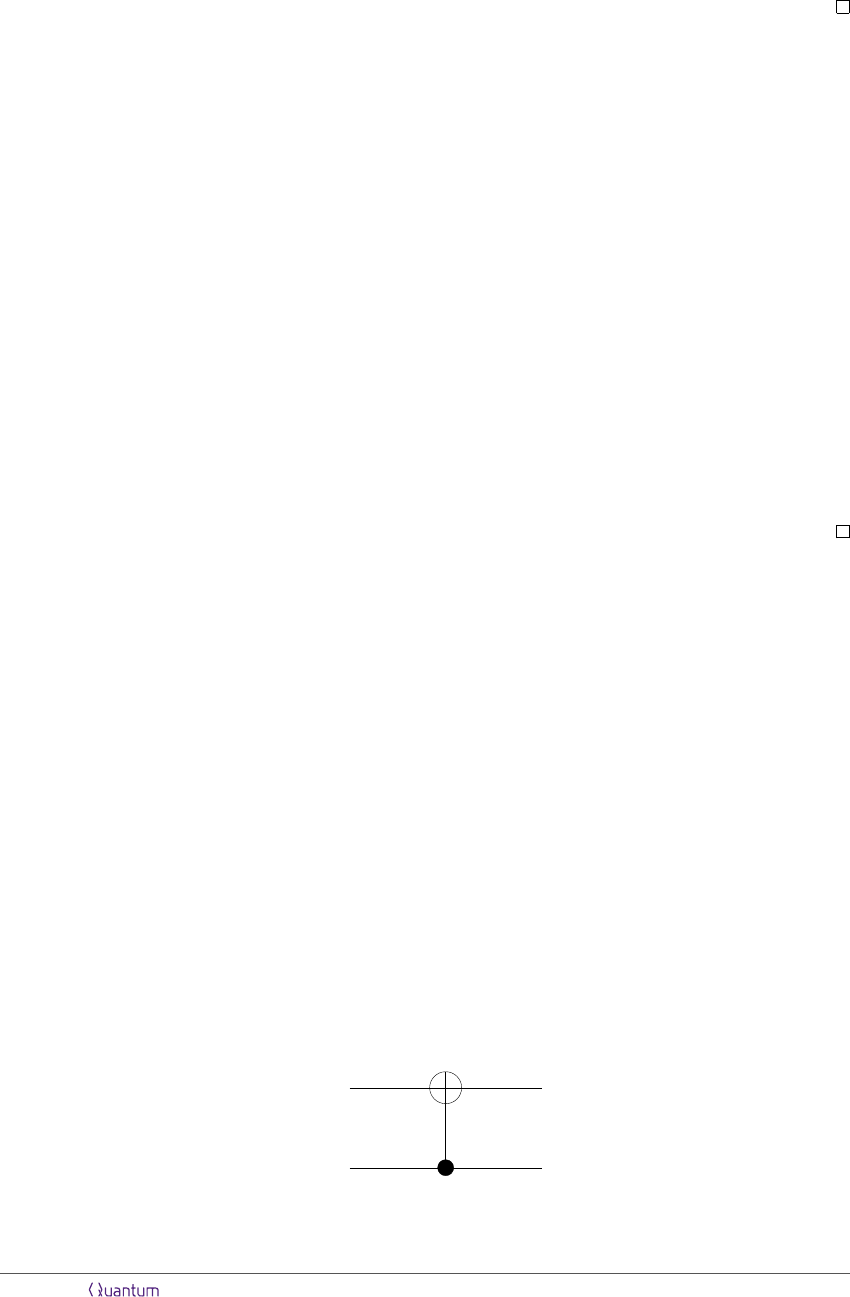original system V produces a new representation V C
d
of G with character
V
. Since we
allow our algorithm to introduce any such workspace, we are in eﬀect allowing it to increase the
multiplicity of each character by a factor of d. Note that this process will never produce irreps
which did not appear in V to begin with. Hence the optimal success probability depends only on
which irreps appear in V , i.e. the set I(V ).
It makes sense that if an algorithm is granted access to more representations to work with, its
success probability cannot decrease. To be more precise, ﬁx t, and let P
opt
(G, V, π, f) denote the
optimal success probability of a t-query algorithm for the symmetric oracle problem (G, V, π, f ).
Lemma 2.4. Suppose π
V
, π
W
are representations of G on the spaces V and W , with I(W ) I(V ).
Then
P
opt
(G, W, π
W
, f) P
opt
(G, V, π
V
, f).
Proof. The basic idea is any t-query algorithm to solve (G, W, π
W
, f) can be extended to produce a
t-query algorithm for (G, V, π
V
, f). Suppose an algorithm A for W uses an N dimensional ancilla
space, i.e. operates on W C
N
. Since I(W ) I(V ), there exists some M so that V C
M
contains a subrepresentation isomorphic to W C
N
. Hence we can write V C
M
= W
0
Y
where W
0
=
W C
N
as CG-modules. Now we claim the initial state, intermediate unitaries, and
POVM for the algorithm A can be extended to an algorithm A
0
acting on V C
M
. The initial
state for A
0
is the vector in W
0
V C
N
corresponding to the initial state for A in W C
N
.
The intermediate unitaries for A
0
act on W
0
according to the unitaries for the algorithm A and
are extended arbitrarily to Y . The measurement operators for A
0
all agree with the measurement
operators for A on W
0
and all but one of the operators act as 0 on the subspace Y . To satisfy the
completeness relation on V C
M
, exactly one of the POVMs should act as the identity on Y (this
modiﬁcation is unimportant since A
0
“takes place” entirely within W
0
). The success probability of
A
0
is equal to that of A.
3 Parallel queries suﬃce
Here we prove Theorem 2.1, namely that the optimal success probability for coset identiﬁcation
can be attained by a parallel (nonadaptive) algorithm. We prove this by showing that any t-query
adaptive algorithm can be converted to a t-query nonadaptive algorithm without aﬀecting the suc-
cess probability. Another way to say this is that every t-query adaptive algorithm can be simulated
by a t-query nonadaptive one. This technique is greatly inspired by the work of Zhandry [Zha15]
who proves this result when G is abelian, and also bears resemblance to the lower bound technique
of Childs, van Dam, Hung and Shparlinski [CvDHS16], where the special case of polynomial inter-
Let π : G U(V ) be a unitary representation of G. Let CG denote the group algebra of
G. Each h G acts on CG by left multiplication, an operator we denote L
h
. We will use the
controlled multiplication operator ([DBCW
+
02]) deﬁned on V CG by
CM|v, gi = |π(g
1
)v, gi.
This deﬁnes a unitary operator and is a generalization of the standard CNOT gate (take G = Z
2
and V = CZ
2
). As such we draw it using circuit diagrams as in section 3.
V
CG
Figure 1: Notation for the controlled multiplication gate CM .
Accepted in Quantum 2021-03-03, click title to verify. Published under CC-BY 4.0. 7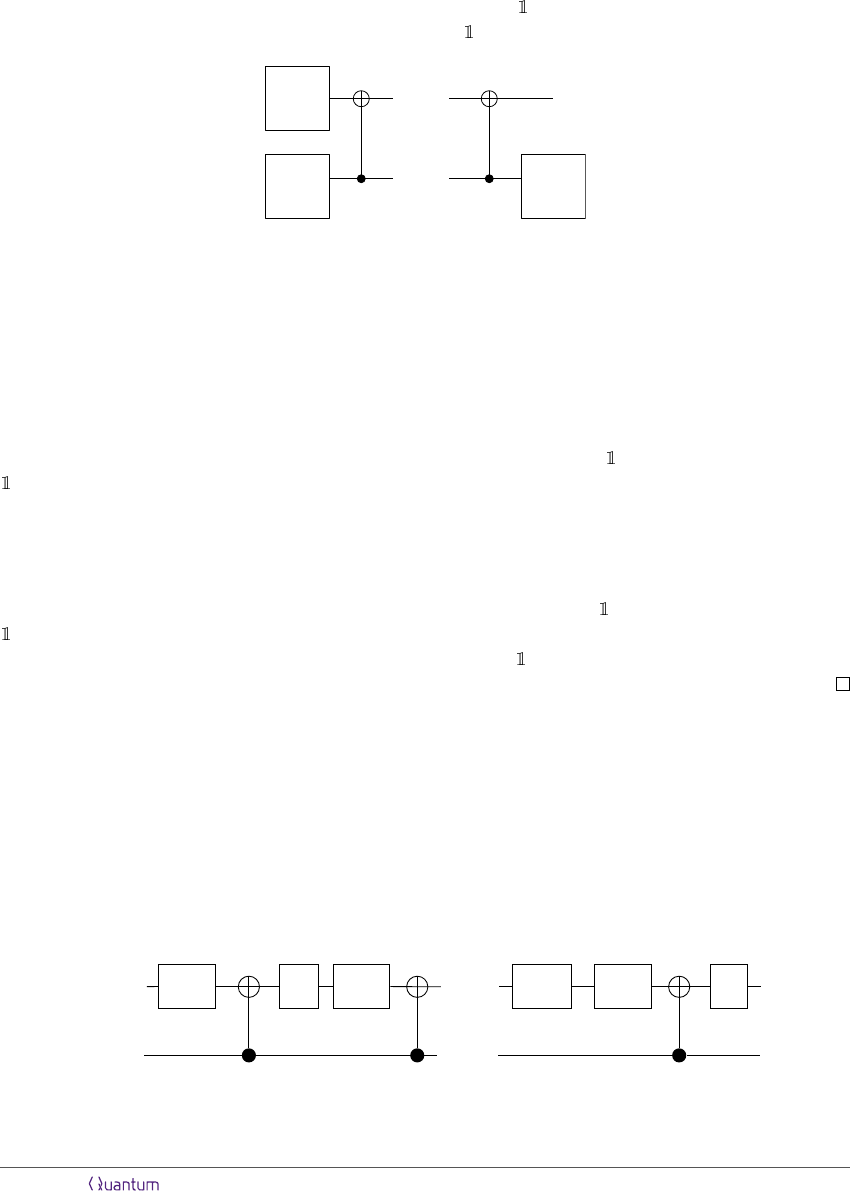There are two G-actions on V CG we use, one given by π(h) L
h
and the other I L
h
. Our
ﬁrst observation is that CM intertwines these actions.
Lemma 3.1. The controlled multiplication operator satisﬁes
CM(π(h) L
h
) = (I L
h
)CM.
The proof follows by applying both sides to a vector |v, gi and using the deﬁnition of CM. The
representation obtained by letting each h G act by the identity on V is a direct sum of dim V
many copies of the trivial reprseentation, so we denote it
dim V
. The lemma allows us to in-
terpret CM as a CG-module isomorphism V CG
dim V
CG. In pictures the lemma reads:
π(h)
L
h
L
h
=
Figure 2: Lemma 3.1 in pictures.
The next property is crucial for our parallelization argument. Recall that if W is a CG-module
then I(W ) denotes the set of irreducible characters of G which appear in W .
Lemma 3.2. Suppose W is a subrepresentation of CG. Then there is a subrepresentation Y of CG
such that the image of V W under CM is contained in V Y and Y satisﬁes I(Y ) = I(V W ).
Proof. By Lemma 3.1 CM is a CG-module isomorphism V CG
dim V
CG where V and
dim V
have the same underlying vector space. Let Z denote the image of V W under CM.
Then CM restricts to a CG-module isomorphism V W Z. Next let Y be the submodule of
CG which contains each irreducible of I(Z) with maximal multiplicity (so if χ appears in Y then χ
appears with multiplicity χ(e)). Now Z
=
V W as CG-modules so in particular I(Z) = I(V W).
Hence also I(Y ) = I(V W ).
It remains to prove Z V Y . Indeed, in the CG-module
dim V
CG the subspace
dim V
Y is the maximal subrepresentation containing only irreducibles in I(V W ). As noted
Z contains only irreducibles in I(V W ) so therefore Z
dim V
Y , which is the same vector
space as V Y .
Now suppose (G, V, π, f) is an instance of coset identiﬁcation and A =
(N, |ψi, {U
1
, . . . , U
t
}, {E
x
}) is a t-query adaptive algorithm to evaluate the homomorphism
f. First, by replacing π with π I if necessary, we may assume that the algorithm does not use a
workspace, that is N = 1. We will describe a new adaptive algorithm A
0
which is a modiﬁcation
of A as follows. We introduce a new workspace which is a copy of CG. The new intermediate
unitaries are (U
1
I)CM, (U
2
I)CM, . . . , (U
t
I)CM. The input state is |ψi |ηi where η is
the equal superposition state in CG. When the oracle is hiding the unitary π(a) this produces the
following state:
|ψi
|ηi
π(a)
U
1
π(a)
. . .
U
t1
π(a)
U
t
Figure 3: Pre-measurement state for A
0
.
Accepted in Quantum 2021-03-03, click title to verify. Published under CC-BY 4.0. 8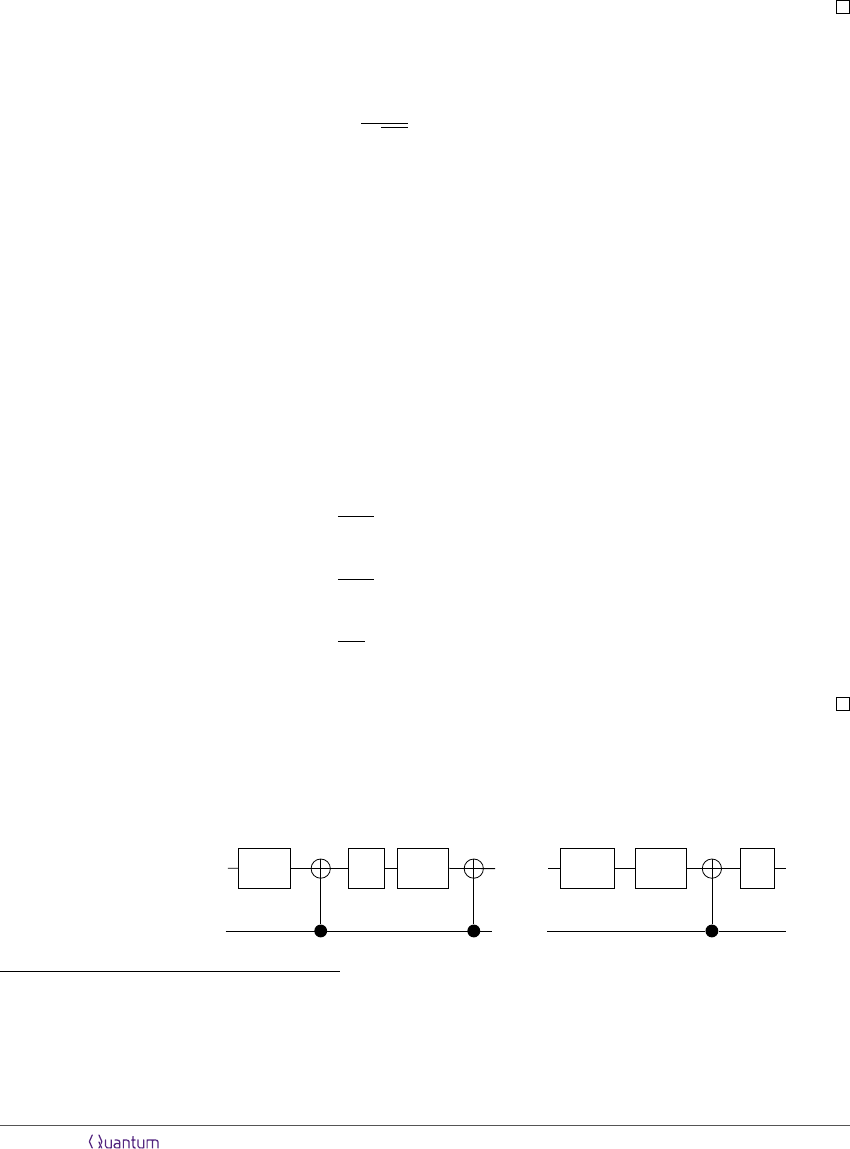Next measurement is performed: ﬁrst the second register is measured in the standard basis of
CG. Then the original POVM is applied to the ﬁrst register. The result of these two measurements
will be a pair (g, x); the ﬁnal output of the algorithm is gx.
3
Lemma 3.3. The algorithm A
0
succeeds with the same success probability as A.
Lemma 3.4. The algorithm A
0
can be simulated by a t-query parallel query algorithm.
Proof of Theorem 2.1 from Lemmas 3.3 and 3.4. By the two lemmas, given any t-query adaptive
algorithm A which solves coset identiﬁcation with probability P , there exists a t-query parallel
query algorithm which succeeds with the same probability.
Proof of Lemma 3.3. Consider the pre-measurement state for A
0
given that the hidden group ele-
ment is a G. It can be written
|ψ
A
0
a
i =
1
p
|G|
X
gG
|ψ
A
g
1
a
i |gi.
If the ﬁrst measurement reads g then the state collapses to |ψ
A
g
1
a
i|gi. If the second measurement
is now performed, the result will read f(g
1
a) with the same probability that the algorithm A
would read this result given that the oracle was hiding g
1
a. The algorithm then classically
converts the result to gf (g
1
a) which is equal to f(a) since f is a left G-set map. So the following
conditional probabilities are equal:
P (A
0
outputs f(a) | a is hidden , ﬁrst measurement result is g)
= P (A outputs f(g
1
(a)) | g
1
a is hidden ).
Denote these probabilities by P
A
0
(f(a) | a, g) and P
A
(f(g
1
a) | g
1
a) respectively. Since the prob-
ability that the ﬁrst measurement of A
0
reads g is 1/|G| for all G and g is sampled independently
of a, we compute the average case success probability by
P
succ
(A
0
) =
1
|G|
2
X
gG
X
aG
P
A
0
(f(a) | a, g)
=
1
|G|
2
X
gG
X
aG
P
A
(f(g
1
a) | g
1
a)
=
1
|G|
X
gG
P
succ
(A) = P
succ
(A).
Proof of Lemma 3.4. We rewrite the pre-measurement state of A
0
expressed by Figure 3 using
Lemma 3.1. Denote the state that results when the hidden element is a G by |ψ
A
0
a
i. We apply
Lemma 3.1 diagrammatically from left to right:
3
Formally the algorithm A
0
is given by
A
0
= (|G|, |ψ, ηi, {U
1
I CM, . . . , U
t
I CM}, {E
0
x
=
X
gG
E
g
1
x
|gihg|}).
Accepted in Quantum 2021-03-03, click title to verify. Published under CC-BY 4.0. 9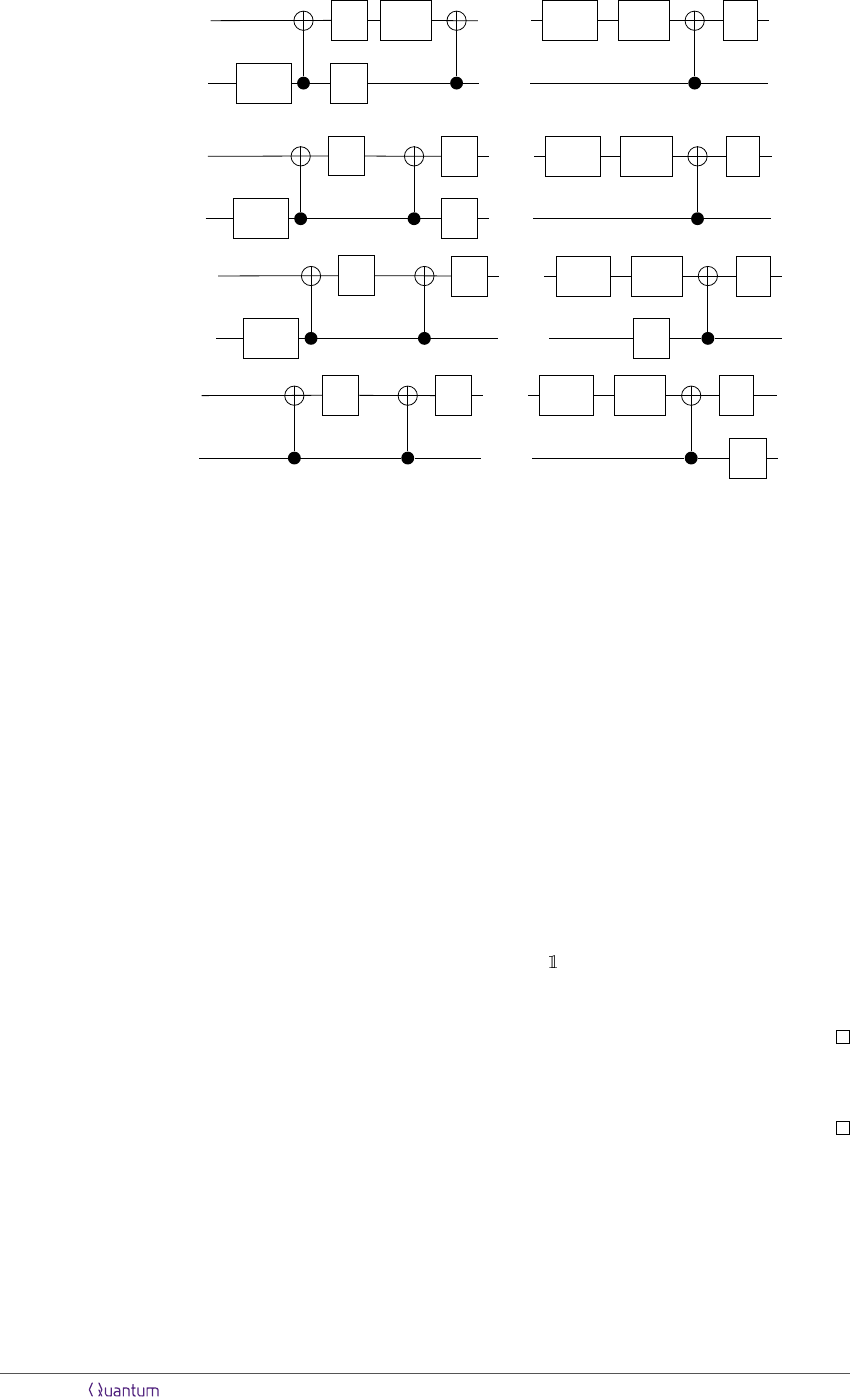|ψi
|ηi
=
L
a
1
U
1
π(a)
L
a
. . .
U
t1
π(a)
U
t
|ψi
|ηi
=
L
a
1
U
1
L
a
. . .
U
t1
π(a)
U
t
U
2
|ψi
|ηi
· · · =
L
a
1
U
1
. . .
U
t1
π(a)
U
t
U
2
L
a
|ψi
|ηi
=
U
1
. . .
U
t1
π(a)
U
t
U
2
L
a
In the last step, in addition to applying Lemma 3.1 at the right of the diagram, we used the
fact that L
a
1
|ηi = |ηi. In formulas we have
|ψ
A
0
a
i = (I L
a
)
(U
t
I) CM ··· (U
1
I) CM
|ψ, ηi.
Therefore we have converted this algorithm to a single-query algorithm using the oracle I L
a
with initial state U |ψ, ηi where U = (U
t
I) CM ··· (U
1
I) CM.
Claim. The image of V C|ηi under U is contained in V Y where Y CG is a submodule
satisfying I(Y ) = I(V
t
).
This is readily proved by induction and Lemma 3.2. For instance, by Lemma 3.2 the image of
V C|ηi under CM is contained in V Y
1
where Y
1
is a submodule with I(Y
1
) = I(V ). The next
part of U is U
1
I which sends V Y
1
to itself. Now another CM is applied and by Lemma 3.2
this sends V Y
1
to V Y
2
where I(Y
2
) = I(V Y
1
) = I(V
2
).
Therefore the inital state U|ψ, ηi belongs to the subspace V Y , which means that the algorithm
A
0
may be simulated by a single query algorithm to the oracle I L
a
acting on the subspace V Y .
Note that the irreducibles appearing in this subspace are I(
dim V
Y ) = I(Y ) = I(V
t
).
Hence Lemma 2.3 implies there exists a single-query algorithm using the representation V
t
which
achieves the same success probability as A
0
. As noted in Lemma 2.2 this is the same as a t-query
parallel algorithm using the representation V . This concludes the proof of Lemma 3.4.
Corollary 3.5. The optimal t-query success probability for an algorithm solving an instance of
coset identiﬁcation (G, V, π, f) is equal to the optimal single-query success probability achievable
solving the instance (G, V
t
, π
t
, f).
4 Application to symmetric oracle identiﬁcation
hiding a group element a G, determine a exactly. This is the special case of coset identiﬁ-
cation in which H = {e}. Thus an instance of this problem is determined by a ﬁnite group G
and a (ﬁnite-dim) unitary representation π : G U (V ). The following theorem computes the
Accepted in Quantum 2021-03-03, click title to verify. Published under CC-BY 4.0. 10success probability of a single-query algorithm and is proved by Bucicovschi, Copeland, Meyer and
Pommersheim:
Theorem 4.1. ([BCMP16], Theorem 1) Suppose G is a ﬁnite group and π : G U(V ) a unitary
representation of G. Then an optimal single-query algorithm to solve symmetric oracle discrimi-
nation succeeds with probability
P
opt
=
d
V
|G|
where
d
V
=
X
χI(V )
χ(e)
2
.
The result of the previous section tells us that parallel algorithms are optimal for symmetric
oracle discrimination.
Theorem 4.2. Suppose G is a ﬁnite group and π : G U(V ) a unitary representation of G. Then
an optimal t-query algorithm to solve symmetric oracle discrimination succeeds with probability
P
opt
=
d
V
t
|G|
where
d
V
t
=
X
χI(V
t
)
χ(e)
2
.
Proof. Theorem 2.1 tells us that a t-query parallel algorithm achieves the optimal success proba-
bility. As noted this is equivalent to a single-query algorithm using the representation π
t
: G
U(V
t
). Now apply Theorem 4.1.
To express the exact and bounded error query complexity of symmetric oracle discrimination
we’re compelled to make the following deﬁnitions.
Let V denote a CG-module. The quantum base size, denoted γ(V ), is the minimum t for which
every irrep of G appears in V
t
. If no such t exists then γ(V ) = . The bounded error quantum
base size, denoted γ
bdd
(V ) is the minimum t for which
1
|G|
X
χI(V
t
)
χ(e)
2
2/3.
If (G, V, π) is a case of symmetric oracle discrimination then by Theorem 4.2 the number of
queries needed to produce a probability 1 algorithm is γ(V ). That is, the exact quantum query
complexity of the problem is equal to the quantum base size of V . Similarly the bounded error
query complexity is γ
bdd
(V ).
It may happen that one of these quantities is inﬁnite. However when V is a faithful represen-
tation then a classical result attributed to Brauer and Burnside ([Isa76], Theorem 4.3) guarantees
that every irrep of G appears in one of the tensor powers V
0
, V, V
2
, . . . , V
m1
where m is the
number of distinct values of the character of V . If V contains a copy of the trivial representation,
then we can say that every irrep of G is contained in some tensor power V
t
for some t. Hence in
this case (with V faithful and containing a copy of the trivial irrep) both γ(V ) and γ
bdd
(V ) are
ﬁnite.
In particular, this occurs whenever we “quantize” a classical symmetric oracle discrimina-
tion problem. This is the learning problem speciﬁed by a ﬁnite set and a homomorphism
G Sym(Ω). A query to an oracle hiding a G consists of inputting ω and receiving
Accepted in Quantum 2021-03-03, click title to verify. Published under CC-BY 4.0. 11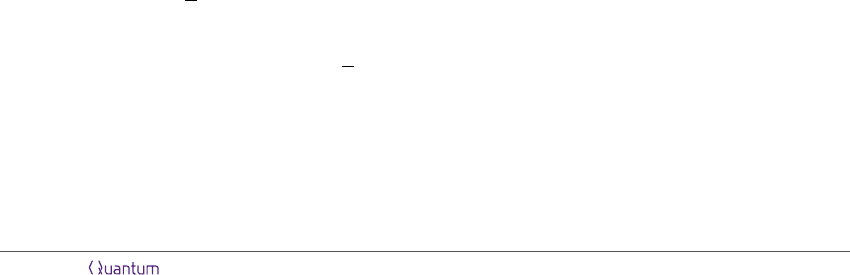a · ω. The learner must determine the hidden group element (or permutation) a. The quantized
learning problem uses the homomorphism G U(CΩ) sending elements of G to permutation
matrices. (Such a representation is called a permutation representation.) Then the quantized
learning problem is faithful if the original problem is faithful and the CG-module contains a copy
of the trivial representation, namely span{
P
ω
|ωi}.
This is precisely the situation we would like to study because we can compare the classical and
quantum query complexity. Classically the exact and bounded error query complexities are equal,
since if a classical algorithm does not use enough queries to identify the hidden permutation with
certainty then it must make a guess between at least 2 equally likely permutations which behave
the same on all the queries that were used, resulting in a success rate of at most 1/2.
Suppose = {1, . . . , n} hosts the deﬁning permutation representation of G = S
n
. Then
n 1 queries are required to determine a hidden permutation σ.
If we take the same action but restrict the group to A
n
S
n
then we need n 2 queries to
determine a hidden element σ A
n
.
Consider the action of the dihedral group D
n
on the set of vertices of an n-gon. Then 2
queries are required to determine a hidden group element.
In general the classical query complexity is a well-known invariant of a permutation group G
denoted b(G) called the minimal base size or just base size of G [LS:02]. It may be deﬁned to be the
length of the smallest tuple (ω
1
, . . . , ω
t
)
t
with the property that (g·ω
1
, . . . , g·ω
t
) = (ω
1
, . . . , ω
t
)
if and only if g = 1. From the deﬁnition it is clear that the base size agrees with the non-adaptive
classical query complexity of the problem. In fact, it is also equal to the adaptive query complexity,
since if a sequence of adaptive guesses (ω
1
, . . . , ω
t
) suﬃces to identify a particular hidden g G,
then the same sequence of guesses works for every element of the group. This means any optimal
algorithm may be implemented non-adaptively. Thus the classical query complexity of symmetric
oracle discrimination of G Sym(Ω) is the base size of G and the quantum exact (bounded error)
query complexity is the (bounded error) quantum base size. We are naturally led to a broad
group theoretic problem:
Question. What are the relationships between b(G), γ(CΩ) and γ
bdd
(CΩ)?
We are not aware of any direct comparison of these quantities in the group theory literature.
Here we only compute the various quantities for some special cases. We saw earlier that b(S
n
) =
n 1. We will prove
Theorem 4.3. Let γ, γ
bdd
denote the quantum base sizes for S
n
acting on {1, . . . , n}. Then
1. γ = n 1 queries are necessary and suﬃcient for exact learning.
2. γ
bdd
= n 2
n + Θ(n
1/6
) queries are necessary and suﬃcient to succeed with probability
2/3.
3. In fact, for any (0, 1), n 2
n + Θ(n
1/6
) queries are necessary and suﬃcient to succeed
with probability 1 .
Proof. Recall that the irreducible characters of S
n
are parametrized by partitions of n which can
be written either as a sequnce [λ
1
, . . . , λ
n
] or as a Young diagram with n total boxes and λ
i
Accepted in Quantum 2021-03-03, click title to verify. Published under CC-BY 4.0. 12boxes in the ith row. Let V = C{1, . . . , n} denote the CG-module corresponding to the deﬁning
permutation representation of S
n
. Then V decomposes as a sum of two irreducibles:
V = V
[n]
V
[n1,1]
.
We note that V
[n]
is the trivial representation. A well-known rule says that if V
λ
is a simple
representation corresponding to the Young diagram λ then the irreps appearing in V V
λ
I(V V
λ
) = {V
µ
| µ λ
±
}.
where λ
±
is the set of Young diagrams obtained from λ by adding then removing a box from
lambda. In particular, this shows by induction that
I(V
t
) = {V
µ
| µ has at least n t columns}.
We see that n 1 queries are required until every irreducible is contained in V
t
(in particular,
the sign representation corresponding to the partition [1
n
] = [1, 1, . . . , 1] is not included in V
t
unless t n 1). This proves part (1) of the theorem.
To prove part (2) we must examine more closely the set I
t
= I(V
t
) consisting of all partitions
with at least n i columns (i.e. λ
1
n i). We are interested in the sum
d
t
:= d
V
t
=
X
χI(V
t
)
χ(e)
2
.
It is well known that if χ is an irrep corresponding to the Young diagram λ then χ(e) is equal
to the number of standard tableaux of shape λ ([Sag01], Theorem 2.5.2). Hence χ(e)
2
is equal
to the number of pairs of standard tableaux of shape λ. Now by the Robinson-Schensted corre-
spondence, the sum above is equal to the number of sequences of the numbers {1, . . . , n} whose
longest increasing subsequence is at least n t (see e.g. [Sag01], Theorem 3.3.2). Next, a deep
result of Baik, Deift and Johannson [BDJ99] identiﬁes the distribution of the l
n
, the length of
the longest increasing subsequence of a random permutation of n elements, as the Tracy-Widom
distribution (which also governs the largest eigenvalue of a random Hermitian matrix) of mean
2
n and standard deviation n
1/6
. In particular, Theorem 1.1 of [BDJ99] asserts that if F (x) is
the cumulative distribution function for the Tracy-Widom distribution, then
lim
n→∞
P rob
l
n
2
n
n
1/6
x
= F (x)
Let c be any real number. If we use t = n 2
n + cn
1/6
queries, then our success probability will
be
P rob(l
n
n t) = 1 P rob(l
n
< 2
n cn
1/6
) = 1 P rob
l
n
2
n
n
1/6
< c
1 F (c)
Thus for any (0, 1), if we wish to succeed with probability 1 , it will be necessary and
suﬃcient to use t = n 2
n + cn
1/6
queries, where c = F
1
() (for n suﬃciently large).
Here is the analogous result for identifying an element of the alternating group.
Theorem 4.4. Consider the standard action of A
n
acting on {1, . . . , n}. Then the quantum base
sizes are given as follows.
1. γ = n d
ne are necessary for exact learning.
2. γ
bdd
= n2
n+Θ(n
1/6
) are necessary and suﬃcient to succeed with probability 2/3. In fact,
for any (0, 1), n 2
n + Θ(n
1/6
) are necessary and suﬃcient to succeed with probability
1 .
Accepted in Quantum 2021-03-03, click title to verify. Published under CC-BY 4.0. 13Proof. Recall the following facts about the representation theory of A
n
. The conjugate of a par-
tition λ is the partition λ
obtained by swapping the rows and columns of λ; in other words
λ
= (λ
1
, λ
2
, . . . ) where λ
i
= the number of boxes in the ith column of λ. For each partition λ of
n that is not self-conjugate, i.e. λ 6= λ
, the restriction of V
λ
to A
n
is an irreducible representation
W
λ
of A
n
. Also, W
λ
= W
λ
. For self conjugate λ, the representation V
λ
breaks up into two
distinct irreducible representations W
+
λ
and W
λ
of equal dimension.
Recall from the previous proof that after t queries, we get copies of all the V
λ
such that
λ
1
n t. Observe that for any partition λ, we must have either λ
1
d
ne or λ
1
d
ne.
(If both fail, the partition ﬁts into a square of side length d
ne 1, which contains fewer than n
boxes.) It follows that after t = n d
ne queries, for any λ, we have picked up a copy of V
λ
or
V
λ
. Hence we have every irreducible representation of A
n
. Therefore, n d
ne queries